# Gray Trap 2”

Blueish grey, this rock pops! Dresser trap is commonly referred to as North America’s strongest rock. Used in landscaping, road construction, and making the great american railways. Get some today for any of your needs.

• Used by NASA for test drilling (True story)
• Our largest Dresser Trap
• Looks great everywhere
• Classic blue grey never out of style
• Float in the vacuum of space
If you need help finding out your total cubic yardage use one of our calculators below!
Aerial Map Calculator
Area Totals
Current Area
0 ft2
Total Area
0 ft2
Total Measurement: 0 cubic yards
Total Price: \$0
Square Footage Calculator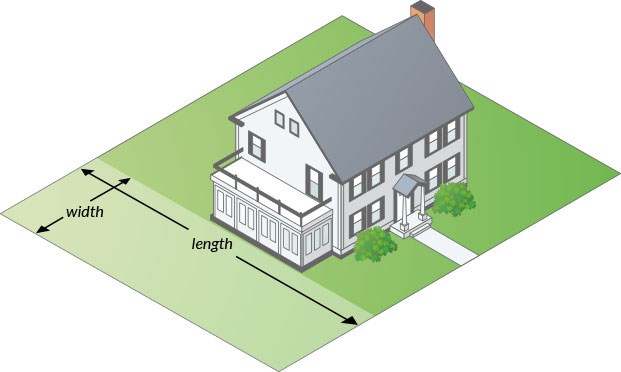#### How to measure your area.

• Area 1
• Area 1
• Area 1
Total Measurement: 0 cubic yards
Total Price: \$0

### How To Measure

#### Square or Rectangle

Measure the Length & Width and multiply.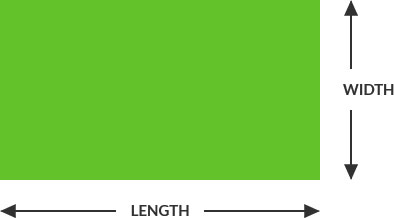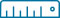Length x Width = Area
(12’ x 24’ = 288 ft2)

#### Right Triangle

Multiply the two sides that contact the right angle and divide by two.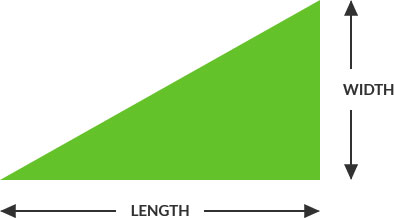Length x Width / 2 = Area
(12’ x 24’ = 288 ÷ 2 = 144 ft2)

#### Circular

Multiply the radius with the radius then multiply by 3.14. Radius is a straight line from the center of a circle to the outside of the circle.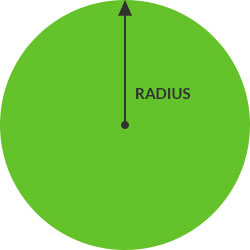##### Full Circle:(6’ x 6’ = 36 x 3.14 = 113 ft2)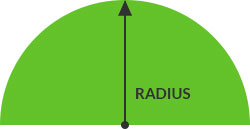##### Half Circle:Divide full circle radius by 2
(6’ x 6’ = 36 x 3.14 = 113 / 2 = 56.5 ft2)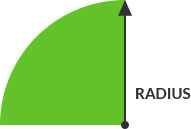##### Quarter Circle:Divide full circle radius by 4
(6’ x 6’ = 36 x 3.14 = 113 / 4 = 28.25 ft2)